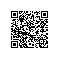# PHP字符串函数之 strstr stristr strchr strrchr

• strstr -- 查找字符串的首次出现，返回字符串从第一次出现的位置开始到该字符串的结尾或开始。
• stristr -- strstr 函数的忽略大小写版本
• strchr -- strstr 函数的别名
• strrchr -- 查找字符串的最后一次出现，返回字符串从最后一次出现的位置开始到该字符串的结尾。

## strstr

mixed strstr ( string $haystack , mixed$needle [, bool $before_needle = false ] ) #### 参数说明 haystack 在该字符串中进行查找。 needle 如果 needle 不是一个字符串，那么它将被转换为整型并被视为字符的顺序值来使用。 before_needle 若为 TRUE，strstr() 将返回 needle 在 haystack 中的位置之前的部分。 #### 返回值 成功：返回字符串 needle 之前或之后的一部分 失败：如果没找到 needle，将返回 FALSE。 #### 注意 1. 该函数区分大小写 2. 如果你仅仅想确定 needle 是否存在于 haystack 中，请使用速度更快、耗费内存更少的 strpos() 函数 #### 示例 <?php /*【 needle 为单个字符 】 */$email  = 'name@example.com';
$domain = strstr($email, '@');
echo $domain; // 打印 @example.com$user = strstr($email, '@', true); // 从 PHP 5.3.0 起 echo$user; // 打印 name
?>
<?php
/*【 needle 为数字 】 */
$email = 'name@example.com'; //字母a的 ASCII码为 97$behind = strstr($email, 97); echo$behind; // 打印 ame@example.com

$front = strstr($email, 97, true); // 从 PHP 5.3.0 起
echo $front; // 打印 n ?> <?php /*【 needle 为字符串 】 */$email = 'name@example.com';
$behind = strstr($email, 'ex');
echo $behind; // 打印 example.com$front = strstr($email, 'ex', true); // 从 PHP 5.3.0 起 echo$front; // 打印 name@
*/
?>
<?php
/*【 needle 为字符串 】 */
$email = 'name@example.com';$behind  = strstr($email, 'ab'); echo$behind; // 返回 false

$front = strstr($email, 'ab', true); // 从 PHP 5.3.0 起
echo $front; // 返回 false */ ?> ## stristr strstr() 函数的忽略大小写版本 mixed stristr ( string$haystack , mixed $needle [, bool$before_needle = false ] )

<?php
$email = 'name@example.com';$behind = stristr($email, 'A'); echo$behind; // 打印 ame@example.com

$front = stristr($email, 'A', true); // 从 PHP 5.3.0 起
echo $front; // 打印 n ?> ## strchr strstr() 函数的别名 mixed strchr ( string$haystack , mixed $needle [, bool$before_needle = false ] )

$email = 'name@example.com';$behind = strchr($email, 'a'); echo$behind; // 打印 ame@example.com

$front = strchr($email, 'a', true); // 从 PHP 5.3.0 起
echo $front; // 打印 n ?> ## strrchr 查找字符串的最后一次出现，返回字符串从最后一次出现的位置开始到该字符串的结尾。 mixed strrchr ( string$haystack , mixed $needle ) #### 参数说明 haystack 在该字符串中进行查找。 needle 如果 needle 包含了不止一个字符，那么仅使用第一个字符。该行为不同于 strstr() 如果 needle 不是一个字符串，那么将被转化为整型并被视为字符顺序值。 #### 返回值 成功：返回字符串 needle 之后的一部分 失败：如果没找到 needle，将返回 FALSE。 #### 示例 <?php /*【 needle 为字符 】 */$email  = 'name@example.com';
$behind = strrchr($email, 'a');
echo $behind; // 打印 ample.com ?> /*【 needle 为字符串 】 */$email  = 'name@example.com';
$behind = strrchr($email, 'am');
echo $behind; // 打印 ample.com ?> <?php /*【 needle 为数字 】 */$email  = 'name@example.com';
$behind = strrchr($email, 97);
echo \$behind; // 打印 ample.com
?>使用钉钉扫一扫加入圈子
+ 订阅